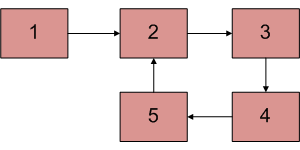# Python Program For Finding The Length Of Loop In Linked List

• Last Updated : 18 May, 2022

Write a function detectAndCountLoop() that checks whether a given Linked List contains loop and if loop is present then returns count of nodes in loop. For example, the loop is present in below-linked list and length of the loop is 4. If the loop is not present, then the function should return 0.Approach:
It is known that Floyd’s Cycle detection algorithm terminates when fast and slow pointers meet at a common point. It is also known that this common point is one of the loop nodes. Store the address of this common point in a pointer variable say (ptr). Then initialize a counter with 1 and start from the common point and keeps on visiting the next node and increasing the counter till the common pointer is reached again.
At that point, the value of the counter will be equal to the length of the loop.
Algorithm:

1. Find the common point in the loop by using the Floyd’s Cycle detection algorithm
2. Store the pointer in a temporary variable and keep a count = 0
3. Traverse the linked list until the same node is reached again and increase the count while moving to next node.
4. Print the count as length of loop

## Python3

 `# Python program to detect a loop and  ` `# find the length of the loop ` `# Node defining class ` `class` `Node: ` `     `  `    ``# Function to make a node ` `    ``def` `__init__(``self``, val): ` `        ``self``.val ``=` `val ` `        ``self``.``next` `=` `None` `     `  `# Linked List defining and loop  ` `# length finding class  ` `class` `LinkedList: ` `     `  `    ``# Function to initialize the  ` `    ``# head of the linked list ` `    ``def` `__init__(``self``): ` `        ``self``.head ``=` `None`         `         `  `    ``# Function to insert a new  ` `    ``# node at the end  ` `    ``def` `AddNode(``self``, val): ` `        ``if` `self``.head ``is` `None``: ` `            ``self``.head ``=` `Node(val) ` `        ``else``: ` `            ``curr ``=` `self``.head ` `            ``while``(curr.``next``): ` `                ``curr ``=` `curr.``next` `            ``curr.``next` `=` `Node(val) ` `     `  `    ``# Function to create a loop in the  ` `    ``# Linked List. This function creates  ` `    ``# a loop by connecting the last node  ` `    ``# to n^th node of the linked list,  ` `    ``# (counting first node as 1) ` `    ``def` `CreateLoop(``self``, n): ` `         `  `        ``# LoopNode is the connecting node to ` `        ``# the last node of linked list ` `        ``LoopNode ``=` `self``.head ` `        ``for` `_ ``in` `range``(``1``, n): ` `            ``LoopNode ``=` `LoopNode.``next` `             `  `        ``# end is the last node of the  ` `        ``# Linked List ` `        ``end ``=` `self``.head ` `        ``while``(end.``next``): ` `            ``end ``=` `end.``next` `             `  `        ``# Creating the loop ` `        ``end.``next` `=` `LoopNode ` `         `  `    ``# Function to detect the loop and return ` `    ``# the length of the loop if the returned  ` `    ``# value is zero, which means that either  ` `    ``# the linked list is empty or the linked  ` `    ``# list doesn't have any loop ` `    ``def` `detectLoop(``self``): ` `         `  `        ``# If linked list is empty then there  ` `        ``# is no loop, so return 0 ` `        ``if` `self``.head ``is` `None``: ` `            ``return` `0` `         `  `        ``# Using Floyd’s Cycle-Finding  ` `        ``# Algorithm/ Slow-Fast Pointer Method ` `        ``slow ``=` `self``.head ` `        ``fast ``=` `self``.head ` ` `  `        ``# To show that both slow and fast  ` `        ``# are at start of the Linked List ` `        ``flag ``=` `0`  `                  `  `        ``while``(slow ``and` `slow.``next` `and` `fast ``and` `              ``fast.``next` `and` `fast.``next``.``next``): ` `            ``if` `slow ``=``=` `fast ``and` `flag !``=` `0``: ` `                 `  `                ``# Means loop is confirmed in the  ` `                ``# Linked List. Now slow and fast  ` `                ``# are both at the same node which ` `                ``# is part of the loop ` `                ``count ``=` `1` `                ``slow ``=` `slow.``next` `                ``while``(slow !``=` `fast): ` `                    ``slow ``=` `slow.``next` `                    ``count ``+``=` `1` `                ``return` `count ` `             `  `            ``slow ``=` `slow.``next` `            ``fast ``=` `fast.``next``.``next` `            ``flag ``=` `1` `        ``return` `0` `# No loop ` `     `  `# Setting up the code ` `# Making a Linked List and  ` `# adding the nodes ` `myLL ``=` `LinkedList() ` `myLL.AddNode(``1``) ` `myLL.AddNode(``2``) ` `myLL.AddNode(``3``) ` `myLL.AddNode(``4``) ` `myLL.AddNode(``5``) ` ` `  `# Creating a loop in the linked List ` `# Loop is created by connecting the  ` `# last node of linked list to n^th node ` `# 1<= n <= len(LinkedList) ` `myLL.CreateLoop(``2``) ` ` `  `# Checking for Loop in the Linked List  ` `# and printing the length of the loop ` `loopLength ``=` `myLL.detectLoop() ` `if` `myLL.head ``is` `None``: ` `    ``print``(``"Linked list is empty"``) ` `else``: ` `    ``print``(``str``(loopLength)) ` `# This code is contributed by _Ashutosh `

Output:

`4`

Complexity Analysis:

• Time complexity:O(n).
Only one traversal of the linked list is needed.
• Auxiliary Space:O(1).
As no extra space is required.

Please refer complete article on Find length of loop in linked list for more details!

My Personal Notes arrow_drop_up
Recommended Articles
Page :